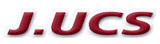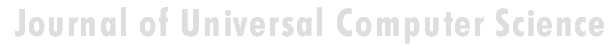Search
 Submission Procedure share: |available in:   PDF (2 MB) PS (909 kB)

get:
 Similar Docs BibTeX Write a comment
get:

DOI:   10.3217/jucs-002-05-0380

### Is Finite Precision Arithmetic Useful For Physics ?

Françoise Chaitin-Chatelin (CERFACS and CEREMADE, University Paris IX Dauphine, France)

Abstract: Both empirical sciences and computations are fundamentally restricted to measurements/computations involving a finite amount of information. These activities deal with the FINITE - some finite precision numbers, coming out from measurements, or from calculations run for some finite amount of time. By way of contrast, as Leibniz expressed it, mathematics is the science of the INFINITE, which contains the concept of continuum. The related concepts of limit points, derivatives and Cantor sets also belong to mathematics, the realm of the infinite, and not to the world of the finite. One is then lead to wonder about the basis for the "unreasonable effectiveness of mathematics in the natural sciences" (Wigner (1960). This puzzling situation gave birth, over the centuries, to a very lively philosophical discussion between mathematicians and physicists. We intend to throw into the debate a few simple examples drawn from practice in numerical analysis as well as in finite precision computations. By means of these examples, we illustrate some aspects of the subtle interplay between the discrete and the continuous, which takes place in Scientific Computing, when solving some equations of Physics.

Is Nature better described by discrete or continuous models at its most intimate level, that is below the atomic level? With the theory of quantum physics, it seems that the question has received a significant push towards a discrete space. However one can argue equally that the time variable in Schrödinger's equation is continuous. We will not get involved in the scholarly dispute between the continuous and the discrete. Instead, we will show on simple examples taken from Scientific Computing, the subtlety of the interplay between the continuous and the discrete, which can take place in computations, be it with finite precision or exact arithmetic.

1.) C. Calude (ed.). The Finite, the Unbounded and the Infinite, Proceedings of the Summer School "Chaitin Complexity and Applications", Mangalia, Romania, 27 June - 6 July, 1995.